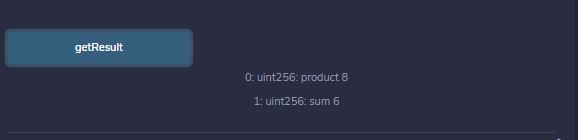Related Articles

# Solidity – View and Pure Functions

The view functions are read-only function, which ensures that state variables cannot be modified after calling them. If the statements which modify state variables, emitting events, creating other contracts, using selfdestruct method, transferring ethers via calls, Calling a function which is not ‘view or pure’, using low-level calls, etc are present in view functions then the compiler throw a warning in such cases. By default, a get method is view function.

Example: In the below example, the contract Test defines a view function to calculate the product and sum of two unsigned integers.

## Solidity

 `// SPDX-License-Identifier: GPL-3.0` `pragma solidity ^0.5.0;` `/// @title A contract for demonstrating view functions` `/// @author Jitendra Gangwar` `/// @notice For now, this contract defining view function to calculate product and sum of two numbers    ` `contract` `Test {` `    ``// Declaring state variables                              ` `    ``uint num1 = 2; ` `    ``uint num2 = 4;`   `   ``function getResult(` `   ``) ``public` `view returns(` `     ``uint product, uint sum){` `      ``product = num1 * num2;` `      ``sum = num1 + num2; ` `   ``}` `}`

Output:Output

The pure functions do not read or modify the state variables, which returns the values only using the parameters passed to the function or local variables present in it. If the statements which read the state variables, access the address or balance, access any global variable block or msg, call a function that is not pure, etc are present in pure functions then the compiler throws a warning in such cases.

Example: In the below example, the contract Test defines a pure function to calculate the product and sum of two numbers.

## Solidity

 `// SPDX-License-Identifier: GPL-3.0` `pragma solidity ^0.5.0;` `/// @title A contract for demonstrating pure functions` `/// @notice For now, this contract defining pure function to calculate product and sum of two numbers    ` `contract` `Test {`   `   ``function getResult(` `   ``) ``public` `pure returns(` `     ``uint product, uint sum){` `      ``uint num1 = 2; ` `      ``uint num2 = 4;` `      ``product = num1 * num2;` `      ``sum = num1 + num2; ` `   ``}` `}`

Output :Output

Whether you're preparing for your first job interview or aiming to upskill in this ever-evolving tech landscape, GeeksforGeeks Courses are your key to success. We provide top-quality content at affordable prices, all geared towards accelerating your growth in a time-bound manner. Join the millions we've already empowered, and we're here to do the same for you. Don't miss out - check it out now!